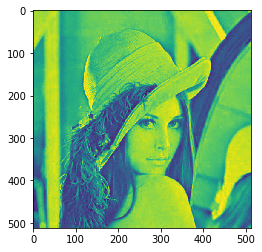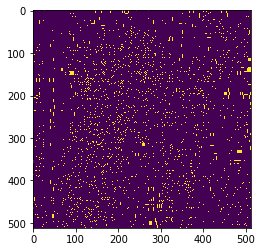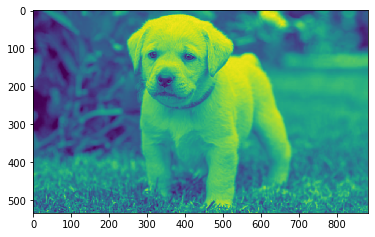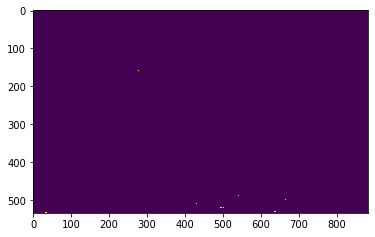GFG App
Open AppBrowser
Continue

# Mahotas – Regional Minima of Image

In this article, we will see how we can get regional minima of images in mahotas. Regional minima is a stricter criterion than the local minima as it takes the whole object into account and not just the neighborhood. Minima are connected components of pixels with a constant intensity value, surrounded by pixels with a higher value.
In this tutorial, we will use the “Lena” image, below is the command to load it.

`mahotas.demos.load('lena')`

Below is the Lena imageIn order to do this we will use mahotas.regmin method
Syntax : mahotas.regmin(img)
Argument : It takes image object as argument
Return : It returns image object

Note: Input image should be filtered or should be loaded as grey
In order to filter the image we will take the image object which is numpy.ndarray and filter it with the help of indexing, below is the command to do this

`image = image[:, :, 0]`

Below is the implementation

## Python3

 `# importing required libraries` `import` `mahotas` `import` `mahotas.demos` `from` `pylab ``import` `gray, imshow, show` `import` `numpy as np` `import` `matplotlib.pyplot as plt` `  `  `# loading image` `img ``=` `mahotas.demos.load(``'lena'``)` `  `  `# filtering image` `img ``=` `img.``max``(``2``)`   `print``(``"Image"``)` `  `  `# showing image` `imshow(img)` `show()`   `# finding regional minima` `new_img ``=` `mahotas.regmin(img)` ` `    `# showing image` `print``(``"Regional Minima"``)` `imshow(new_img)` `show()`

Output :

`Image``Regional Minima`Another example

## Python3

 `# importing required libraries` `import` `mahotas` `import` `numpy as np` `from` `pylab ``import` `gray, imshow, show` `import` `os` `import` `matplotlib.pyplot as plt` ` `  `# loading image` `img ``=` `mahotas.imread(``'dog_image.png'``)`     `# filtering image` `img ``=` `img[:, :, ``0``]` `  `  `print``(``"Image"``)` `  `  `# showing image` `imshow(img)` `show()`   `# finding regional minima` `new_img ``=` `mahotas.regmin(img)` ` `    `# showing image` `print``(``"Regional Minima"``)` `imshow(new_img)` `show()`

Output :

`Image``Regional Minima`My Personal Notes arrow_drop_up